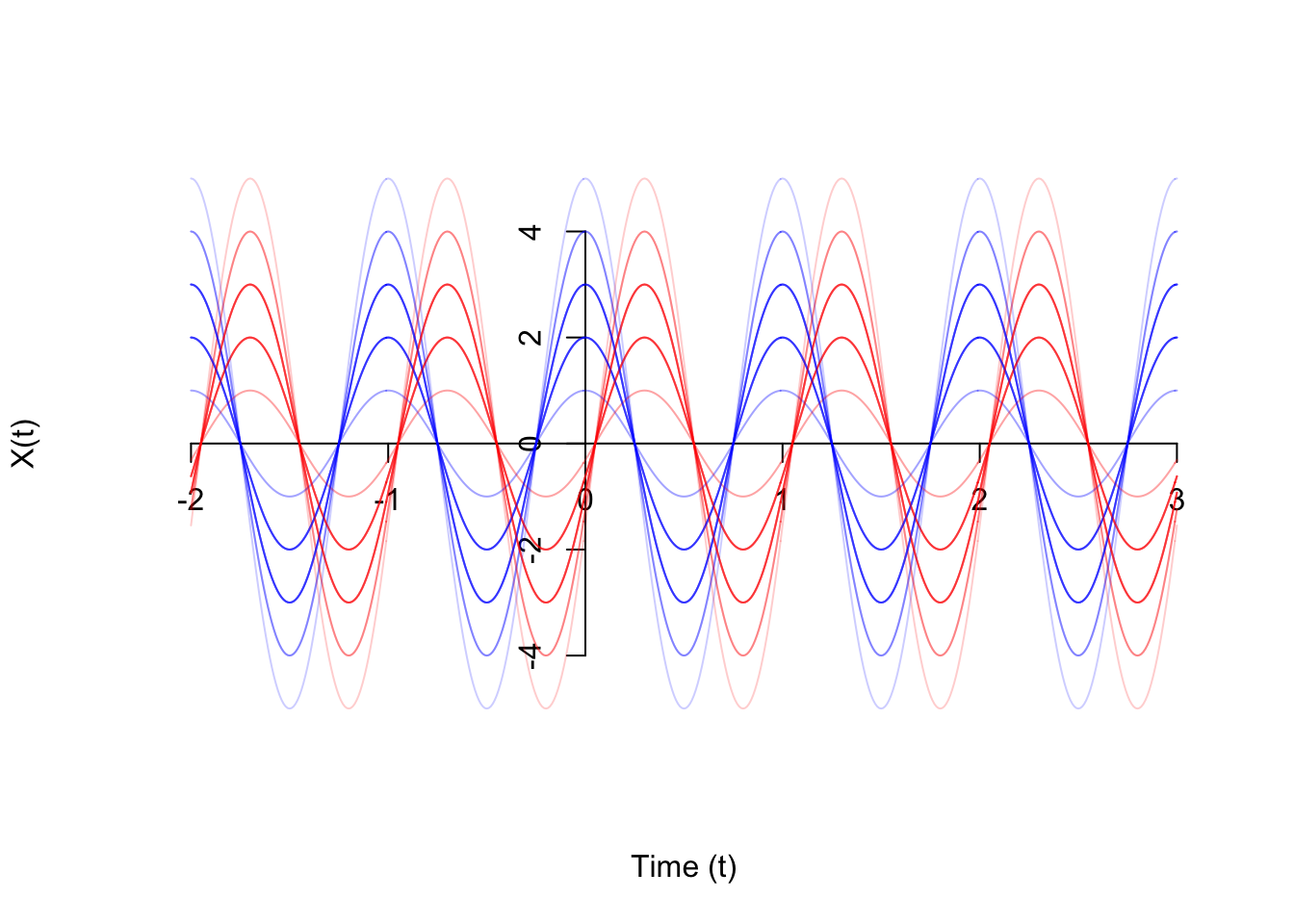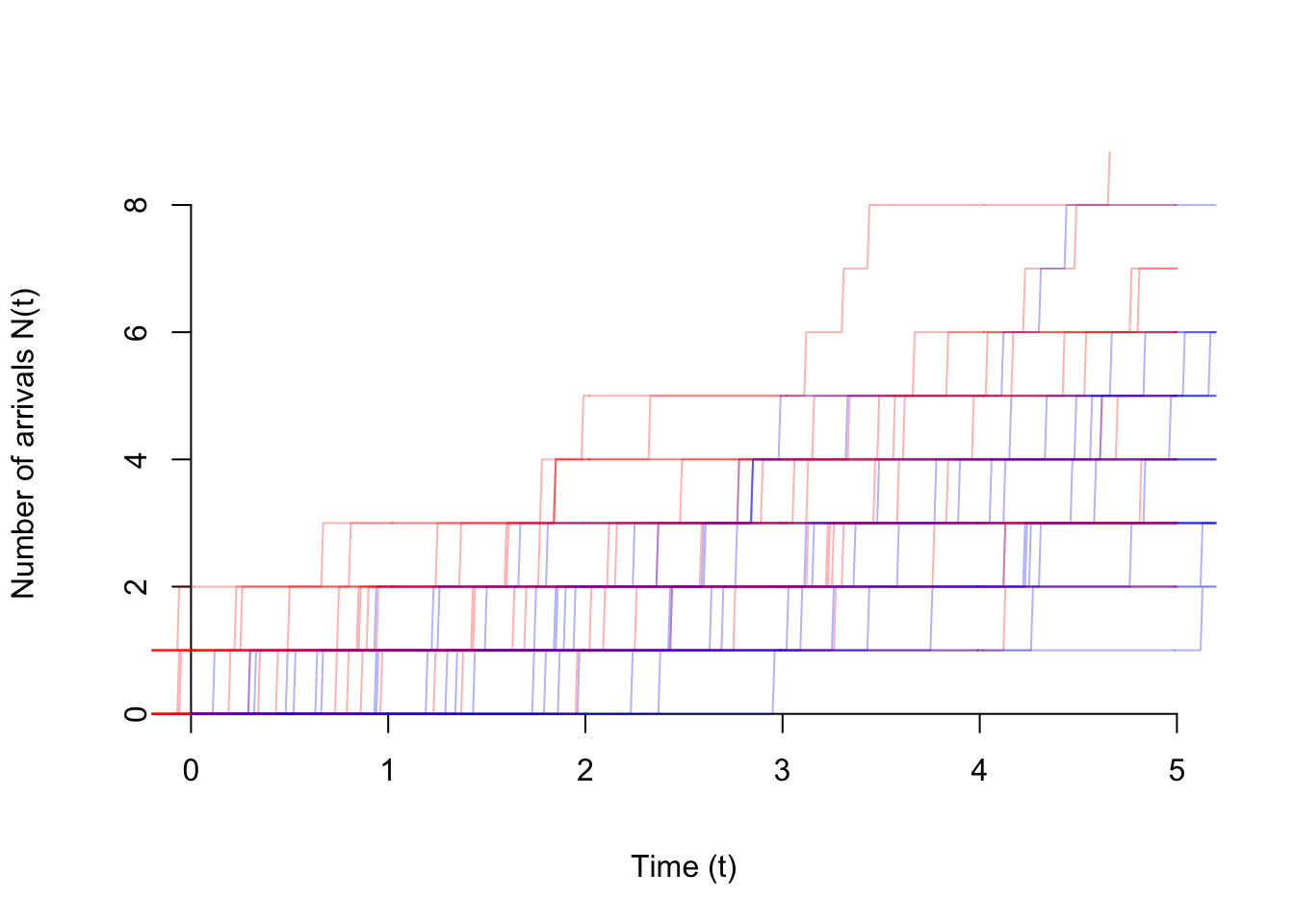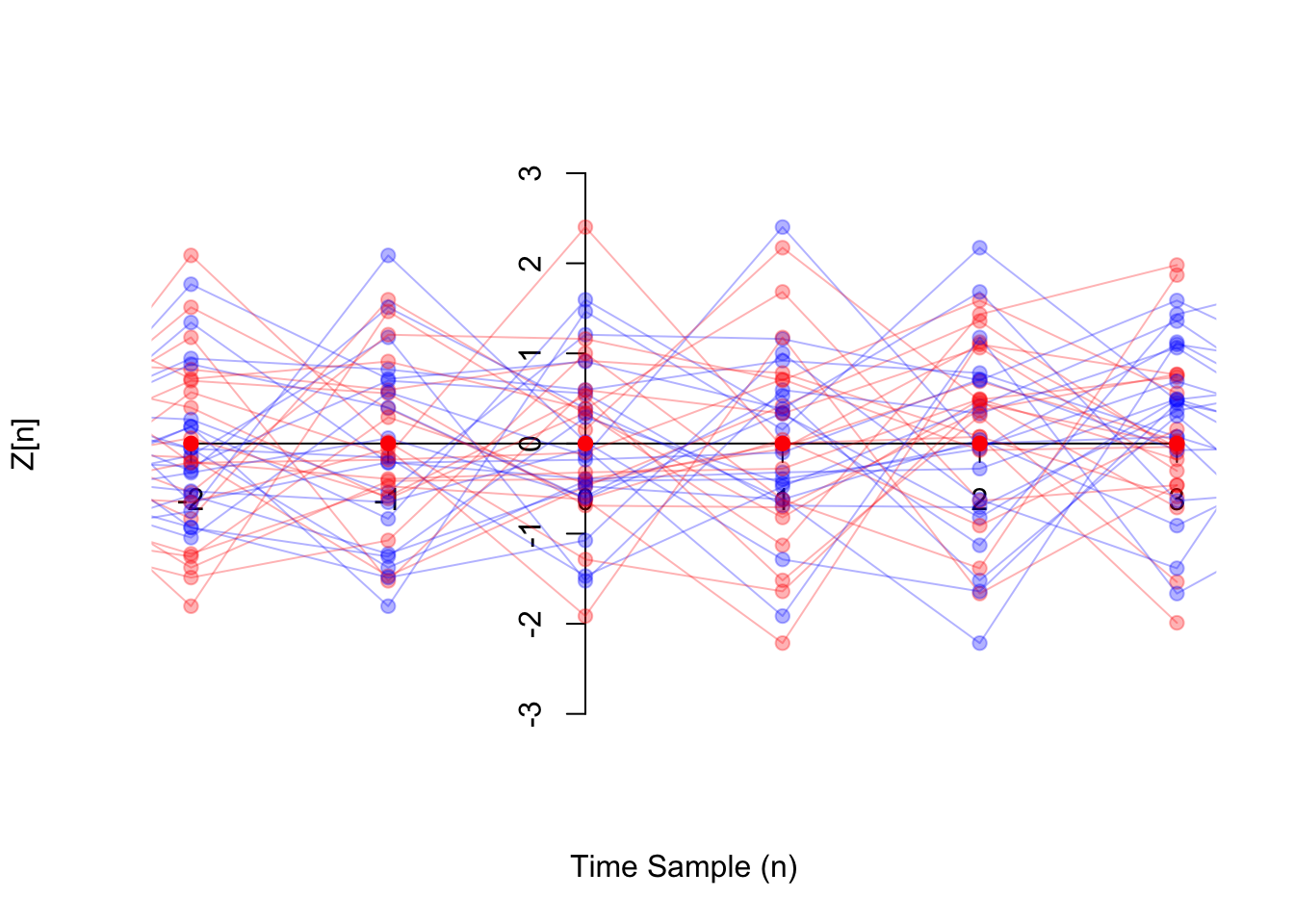# Lesson 53 Stationary Processes

## Motivation

What does it mean for a random process to remain the “same” over time? Obviously, the exact values will be different, since the process is random. The notion of a “stationary process” makes this notion precise.

## Theory

Definition 53.1 (Stationary Process) A process $$\{ X(t) \}$$ is called strict-sense stationary if the process is completely invariant under time shifts. That is, the distribution of $(X(t_1), X(t_2), ..., X(t_n))$ matches the distribution of $(X(t_1 + \tau), X(t_2 + \tau), ..., X(t_n + \tau))$ for any times $$t_1, ..., t_n$$ and any time shift $$\tau$$.

However, we will mostly be concerned with wide-sense stationary processes, which is less restrictive. A random process $$\{ X(t) \}$$ is wide-sense stationary if its mean and autocovariance function are invariant under time shifts. That is:

1. The mean function $$\mu_X(t)$$ is constant. In this case, we will write the mean function as $$\mu_X(t) \equiv \mu_X$$.
2. The autocovariance function $$C_X(s, t)$$ only depends on $$s - t$$, the difference between the times. In this case, we will write the autocovariance function as $$C_X(s, t) = C_X(s - t)$$.

(For a discrete-time process, we require the autocovariance function $$C_X[m, n]$$ to only depend on $$m - n$$, and we write $$C_X[m, n] = C_X[m - n]$$.)

Let’s determine whether some random processes are wide-sense stationary.

Example 53.1 (Random Amplitude Process) Consider the random amplitude process $\begin{equation} X(t) = A\cos(2\pi f t) \tag{50.2} \end{equation}$ introduced in Example 48.1.

In Example 50.1, we showed that its mean function is $\mu_X(t) = 2.5 \cos(2\pi f t).$ This is not constant in $$t$$, so this process cannot be wide-sense stationary. We do not even need to check the autocovariance function.

If we look at a graph of the process, it is clearly not stationary. If we shift the process by any amount that is less than a full period, then the process looks different. Shown on the graph below are 20 realizations of the original process $$\{ X(t) \}$$ in blue, as well as 20 realizations of the time-shifted process $$\{ X(t - 0.3) \}$$. The peaks and troughs are in different places.Example 53.2 (Poisson Process) Consider the Poisson process $$\{ N(t); t \geq 0 \}$$ of rate $$\lambda$$, defined in Example 47.1.

In Example 50.2, we showed that its mean function is $\mu_N(t) = \lambda t.$ This is not constant in $$t$$, so we know that this process cannot be wide-sense stationary. We do not even need to check the autocovariance function.

Again, the fact that the Poisson process is not stationary is immediately apparent from a graph of the process. Shown below are 20 realizations of the original process $$\{ N(t) \}$$ in blue, as well as 20 realizations of the time-shifted process $$\{ N(t - 1) \}$$ in red. The time-shifted process may not even start at 0 at time 0!Example 53.3 (White Noise) Consider the white noise process $$\{ Z[n] \}$$ defined in Example 47.2, which consists of i.i.d. random variables with mean $$\mu = E[Z[n]]$$ and variance $$\sigma^2 \overset{\text{def}}{=} \text{Var}[Z[n]]$$.

In Example 50.3, we showed that its mean function is $\mu_Z[n] \equiv \mu,$ which is constant, so the process could be wide-sense stationary. We need to check its autocovariance function.

In Example 52.3, we showed that its autocovariance function is $C_Z[m, n] = \begin{cases} \sigma^2 & m = n \\ 0 & m \neq n \end{cases}.$

To see that this is a function of $$m - n$$, we rewrite $$m = n$$ as $$m - n = 0$$: $C_Z[m, n] = \begin{cases} \sigma^2 & m - n = 0 \\ 0 & m - n \neq 0 \end{cases}.$

This can be written more compactly using the discrete delta function $$\delta[k]$$, defined as $\delta[k] \overset{def}{=} \begin{cases} 1 & k=0 \\ 0 & k \neq 0 \end{cases}.$ In terms of $$\delta[k]$$, the autocovariance function is simply $C_Z[m, n] = \sigma^2 \delta[m - n].$

The graph below shows white noise $$\{ Z[n] \}$$ in blue and time-shifted white noise $$\{ Z[n-1] \}$$ in red. Visually, it is hard to tell the difference between the two, which is intutively why the process is stationary.Example 53.4 (Random Walk) Consider the random walk process $$\{ X[n]; n\geq 0 \}$$ from Example 47.3.

In Example 50.4, we calculated the mean function to be $\mu[n] = 0.$ This is constant, so the process might be wide-sense stationary. But we also need to check the autocovariance function.

In Example 52.4, we calculated the autocovariance function to be $C[m, n] = \min(m, n) \text{Var}[Z].$ This is not just a function of $$m - n$$, so the process is not wide-sense stationary.

You might ask, “How can we be sure that there isn’t some way to manipulate $$\min(m, n)$$ so that it is a function of $$m-n$$?” We can prove that it is impossible by testing a few pairs of values. For example, $$(m, n) = (3, 2)$$ and $$(m, n) = (6, 5)$$ are two pairs of values that are both separated in time by $$m - n = 1$$ samples. If it were possible to reduce $$C[m, n]$$ to a function $$C[m - n]$$ of the difference only, then $$C[3, 2]$$ would have to equal $$C[6, 5]$$.

However, they are not equal: $$C[3, 2] = 2 \text{Var}[Z]$$, while $$C[6, 5] = 5 \text{Var}[Z]$$.

Example 53.5 Let $$\{ X(t) \}$$ be a continuous-time random process with

• $$E[X(t)] = 2$$ for all $$t$$, and
• $$\text{Cov}[X(s), X(t)] = 5e^{-3(s - t)^2}$$ for all $$s$$ and $$t$$.

The mean function $$\mu_X(t) = E[X(t)]$$ is constant and the autocovariance function $$C_X(s, t) = 5e^{-3(s - t)^2}$$ is a function of $$\tau = s - t$$ only, so the process is stationary.

Since the process is stationary, we can write the mean and autocovariance functions as \begin{align*} \mu_X &= 2 & C_X(\tau) &= 5e^{-3\tau^2}. \end{align*}

## Essential Practice

For these practice questions, you may want to refer to the mean and autocovariance functions you calculated in Lessons 50 and 52.

1. Consider a grain of pollen suspended in water, whose horizontal position can be modeled by Brownian motion $$\{B(t); t \geq 0\}$$ with parameter $$\alpha=4 \text{mm}^2/\text{s}$$, as in Example 49.1. Is $$\{ B(t); t \geq 0 \}$$ wide-sense stationary?

2. Radioactive particles hit a Geiger counter according to a Poisson process at a rate of $$\lambda=0.8$$ particles per second. Let $$\{ N(t); t \geq 0 \}$$ represent this Poisson process.

Define the new process $$\{ D(t); t \geq 3 \}$$ by $D(t) = N(t) - N(t - 3).$ This process represents the number of particles that hit the Geiger counter in the last 3 seconds. Is $$\{ D(t); t \geq 3 \}$$ wide-sense stationary?

3. Consider the moving average process $$\{ X[n] \}$$ of Example 48.2, defined by $X[n] = 0.5 Z[n] + 0.5 Z[n-1],$ where $$\{ Z[n] \}$$ is a sequence of i.i.d. standard normal random variables. Is $$\{ X[n] \}$$ wide-sense stationary?

(Hint: You can write $$m = n + 1$$ as $$m - n = 1$$.)

4. Let $$\Theta$$ be a $$\text{Uniform}(a=-\pi, b=\pi)$$ random variable, and let $$f$$ be a constant. Define the random phase process $$\{ X(t) \}$$ by $X(t) = \cos(2\pi f t + \Theta).$ Is $$\{ X(t) \}$$ wide-sense stationary?